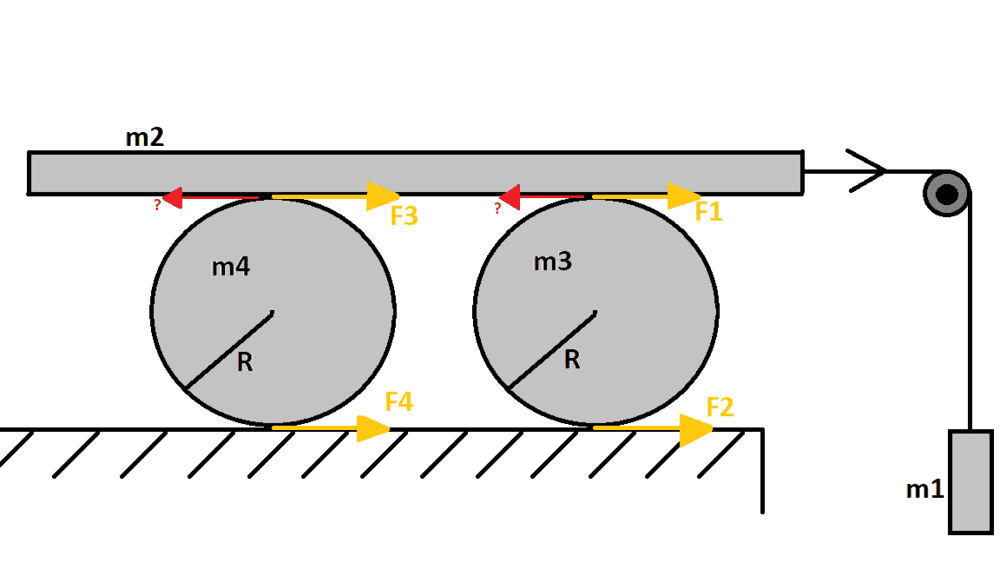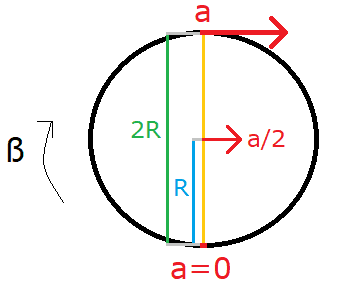Mechanics: Two masses on a pulley causing two cylinders to accelerate

Homework Statement:
The system has no slip
At first the system is resting and at some point we let m1 fall down. I need to find the forces (yellow arrows)
Relevant Equations:
F = m*a
a = R*ß
torque = 1/2*m*R^2Hi! I need help with this problem. m1-2-3-4 and R are given. There is no slip in the system. I have to give F1-2-3-4 in respect of the masses and R.
Here is what I managed to
m1 is easy: m1*a = m1*g - T(tension of the rope)
m2: m2*a = T - (???) <-- I have a problem with this. F1 and F3 is the Force on the wheels "made" by m2. But if m2 rotates the wheels, then m2's acceleration is weakened by the wheels, right? How? I need "new"(red arrows, not given by the teacher) forces acting on m2?

I can calculate the rotational forces on both wheels with this equation: Torque*angular acceleration = R*F(1-3) - R*F(2-4)
I can calculate the horizontal acceleration with this equation: m(3-4)* horizontal acceleration = F(1-3) + F(2-4)
I think the edge of the wheel had the same acceleration as m1 and m2, but I don't know how is it related to the horizontal acceleration.
I found an equation: a = R*angular acceleration, but if I am correct this isn't the acceleration of the rotating outer edge.

Last edited by a moderator:

haruspex
Homework Helper
Gold Member
I need "new"(red arrows, not given by the teacher) forces acting on m2?
What do you know about action and reaction?

•Hohen
What do you know about action and reaction?
Thank you, I know more about it now :)
So if I understood correctly: The red arrows are the reaction forces of f1-3.
m2 imparts f1-3 on the cylinders and the cylinders impart the reaction forces on m2. Can I assume those forces has equal magnitude as f1-3?
m2 ⋅ a = T - f1 - f2 ← Is this equation correct?

haruspex
Homework Helper
Gold Member
Can I assume those forces has equal magnitude as f1-3?
One equals F1 in magnitude, the other F3, yes.
m2 ⋅ a = T - f1 - f2 ← Is this equation correct?
Not quite.. is that what you meant to write or did you make a typo?

I meant to write this.

haruspex
Homework Helper
Gold Member
I meant to write this.
In your diagram the two forces between the rollers and the plank are F1 and F3, not F1 and F2.

Oh sh.. I made a typo indeed, sry :D
So otherwise is it correct?
If it is I know parametrically the acceleration of the plank. The rotational acceleration of the outer edge of the cylinder is the same as the plank's acceleration, right?
I analyzed a similar problem found on the internet. It said the horizontal acceleration of the cylinder is half as the rotational acceleration of the outer edge. Is this true? Can I start to deduce why?

haruspex
Homework Helper
Gold Member
the horizontal acceleration of the cylinder is half as the rotational acceleration of the outer edge
Yes. What is the horizontal acceleration of the point where it touches the ground?

It's always 0.

haruspex
Homework Helper
Gold Member
It's always 0.
Right. So if the horizontal acceleration at the top is a, what is the horizontal acceleration halfway up?

Then half of a but I still don't understand complitly.
I drew another picture:ß is the angular acceleration.
So the equation a = R⋅ß gives me the acceleration in a point with R distance from point 0 (a = 0)?
Is this equation only true on the yellow line?
Is this somehow related to the x and y components of the accelerations?
Let the bottom point be a1, middle a2 and the top a3.
a(vector) = (ay; ax)
ay is centripetal acceleration?
a1 = (ay;0) → |a1| = √(ay^2 + 0^2) → the x component is 0
a2 = (0;ax) → |a1| = √(0^2 + ax^2) → the y component is 0, so the acceleration vector equals the x component
a3 = (ay;ax) → |a1| = √(ay^2 + ax^2) → ???

haruspex
Homework Helper
Gold Member
Then half of a but I still don't understand complitly.
I drew another picture:

View attachment 262537

ß is the angular acceleration.
So the equation a = R⋅ß gives me the acceleration in a point with R distance from point 0 (a = 0)?
Is this equation only true on the yellow line?
Is this somehow related to the x and y components of the accelerations?
Let the bottom point be a1, middle a2 and the top a3.
a(vector) = (ay; ax)
ay is centripetal acceleration?
a1 = (ay;0) → |a1| = √(ay^2 + 0^2) → the x component is 0
a2 = (0;ax) → |a1| = √(0^2 + ax^2) → the y component is 0, so the acceleration vector equals the x component
a3 = (ay;ax) → |a1| = √(ay^2 + ax^2) → ???
A safe way to consider the acceleration of a point on a rigid body is to think of the body's motion as the sum of the motion of its mass centre and its rotation about that centre.
Say a wheel radius r is rolling at speed v and linear acceleration a, and we are interested in the motion of a point at radius x, angle θ 'ahead' of the topmost point.
The angular acceleration of the wheel is a/r and angular velocity v/r. Relative to the centre, the point has tangential acceleration ax/r (forward and down) and centripetal acceleration v2x/r2.
Resolving into horizontal and vertical components, the point has net horizontal acceleration ##a+\frac{ax}r\cos(\theta)-\frac{v^2x}{r^2}\sin(\theta)## and vertical acceleration ##-\frac{ax}r\sin(\theta)-\frac{v^2x}{r^2}\cos(\theta)##.

haruspex
Homework Helper
Gold Member
uh I need time to process this but… I calculated the acceleration.
The result I got is $$a =g⋅ \frac {8(m1)} {8m1+8(m2)+3(m3)+3(m4)}$$
Am I correct?
I attached 2 pictures about how I got this result on paper.

View attachment 262555View attachment 262554
Looks good.

•Hohen
Thank you very much for your help!

One last question: I digged into Lagrangian mechs. a little bit. The second task at this problem to write the Lagrangian equation of the system.
Here is how I calculated:
$$0 = \frac {d} {dt} (\frac {∂L}{∂\dot x}) - \frac {∂L}{∂x}$$

##L = KE-PE##, where ##PE = -m1⋅g##
$$KE = KE_1+KE_2+KE_3+KE_4$$​
##KE = \frac{1}{2} ⋅ m_1 ⋅ \dot x^2 + \frac{1}{2} ⋅ m_2 ⋅ \dot x^2 + (\frac{1}{2} ⋅ m_3 ⋅ (\frac{\dot x}{2})^2 +\frac{1}{2} ⋅ p_3 ⋅ \dot \theta^2)+(\frac{1}{2} ⋅ m_4 ⋅ (\frac{\dot x}{2})^2 +\frac{1}{2} ⋅ p_4 ⋅ \dot \theta^2)##
##KE = \frac{1}{2} ⋅ m_1 ⋅ \dot x^2 + \frac{1}{2} ⋅ m_2 ⋅ \dot x^2 + (\frac{1}{2} ⋅ m_3 ⋅ (\frac{\dot x}{2})^2 +\frac{1}{2} ⋅ (\frac{1}{2} ⋅m_3⋅x _2^2)⋅(\frac{\dot x_2}{2x_2})^2)+(\frac{1}{2} ⋅ m_4 ⋅ (\frac{\dot x}{2})^2 +\frac{1}{2} ⋅ (\frac{1}{2} ⋅m_4⋅x _2^2)⋅(\frac{\dot x_2}{2x_2})^2##
##KE = \frac{1}{2} ⋅ m_1 ⋅ \dot x^2 + \frac{1}{2} ⋅ m_2 ⋅ \dot x^2 + \frac{3}{16} ⋅ m_3 ⋅\dot x^2 +\frac{3}{16} ⋅ m_4 ⋅\dot x^2##

##L=\frac{1}{2} ⋅ m_1 ⋅ \dot x^2 + \frac{1}{2} ⋅ m_2 ⋅ \dot x^2 + \frac{3}{16} ⋅ m_3 ⋅\dot x^2 +\frac{3}{16} ⋅ m_4 ⋅\dot x^2 - m -(-m_1⋅g)##

##\frac {∂L}{∂x}=m_1⋅g##
##\frac {∂L}{∂\dot x} = m_1⋅\dot x + m_2⋅\dot x +\frac{3}{8}⋅m_3⋅\dot x +\frac{3}{8}⋅m_4⋅\dot x##
##\frac {d} {dt} (\frac {∂L}{∂\dot x}) - \frac {∂L}{∂x}= m_1⋅\ddot x + m_2⋅\ddot x +\frac{3}{8}⋅m_3⋅\ddot x +\frac{3}{8}⋅m_4⋅\ddot x - m_1⋅g##

Is this correct? If I hand in the problems, my teacher should accept the last line as the Lagrangian, right?

haruspex
Homework Helper
Gold Member
One last question: I digged into Lagrangian mechs. a little bit. The second task at this problem to write the Lagrangian equation of the system.
Here is how I calculated:
$$0 = \frac {d} {dt} (\frac {∂L}{∂\dot x}) - \frac {∂L}{∂x}$$

##L = KE-PE##, where ##PE = -m1⋅g##
$$KE = KE_1+KE_2+KE_3+KE_4$$​
##KE = \frac{1}{2} ⋅ m_1 ⋅ \dot x^2 + \frac{1}{2} ⋅ m_2 ⋅ \dot x^2 + (\frac{1}{2} ⋅ m_3 ⋅ (\frac{\dot x}{2})^2 +\frac{1}{2} ⋅ p_3 ⋅ \dot \theta^2)+(\frac{1}{2} ⋅ m_4 ⋅ (\frac{\dot x}{2})^2 +\frac{1}{2} ⋅ p_4 ⋅ \dot \theta^2)##
##KE = \frac{1}{2} ⋅ m_1 ⋅ \dot x^2 + \frac{1}{2} ⋅ m_2 ⋅ \dot x^2 + (\frac{1}{2} ⋅ m_3 ⋅ (\frac{\dot x}{2})^2 +\frac{1}{2} ⋅ (\frac{1}{2} ⋅m_3⋅x _2^2)⋅(\frac{\dot x_2}{2x_2})^2)+(\frac{1}{2} ⋅ m_4 ⋅ (\frac{\dot x}{2})^2 +\frac{1}{2} ⋅ (\frac{1}{2} ⋅m_4⋅x _2^2)⋅(\frac{\dot x_2}{2x_2})^2##
##KE = \frac{1}{2} ⋅ m_1 ⋅ \dot x^2 + \frac{1}{2} ⋅ m_2 ⋅ \dot x^2 + \frac{3}{16} ⋅ m_3 ⋅\dot x^2 +\frac{3}{16} ⋅ m_4 ⋅\dot x^2##

##L=\frac{1}{2} ⋅ m_1 ⋅ \dot x^2 + \frac{1}{2} ⋅ m_2 ⋅ \dot x^2 + \frac{3}{16} ⋅ m_3 ⋅\dot x^2 +\frac{3}{16} ⋅ m_4 ⋅\dot x^2 - m -(-m_1⋅g)##

##\frac {∂L}{∂x}=m_1⋅g##
##\frac {∂L}{∂\dot x} = m_1⋅\dot x + m_2⋅\dot x +\frac{3}{8}⋅m_3⋅\dot x +\frac{3}{8}⋅m_4⋅\dot x##
##\frac {d} {dt} (\frac {∂L}{∂\dot x}) - \frac {∂L}{∂x}= m_1⋅\ddot x + m_2⋅\ddot x +\frac{3}{8}⋅m_3⋅\ddot x +\frac{3}{8}⋅m_4⋅\ddot x - m_1⋅g##

Is this correct? If I hand in the problems, my teacher should accept the last line as the Lagrangian, right?

I don't understand your development of ##\frac{1}{2} ⋅ p_3 ⋅ \dot \theta^2## etc. What is x2?
I expected ##p_3=\frac{1}{2}m_3R^2## and ## \dot \theta=\frac{\dot x}{2R}##.